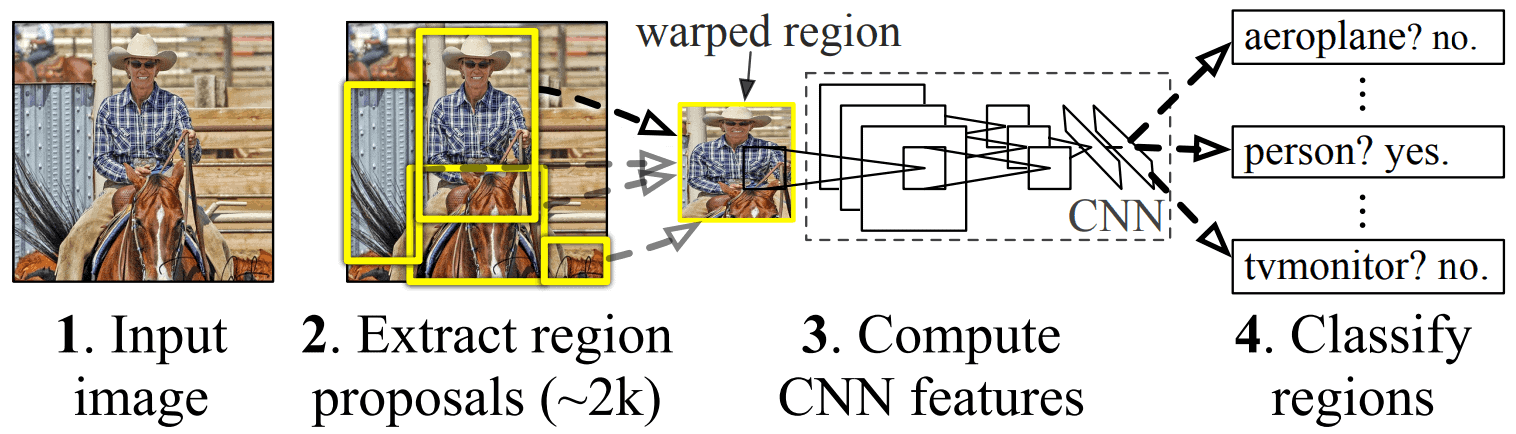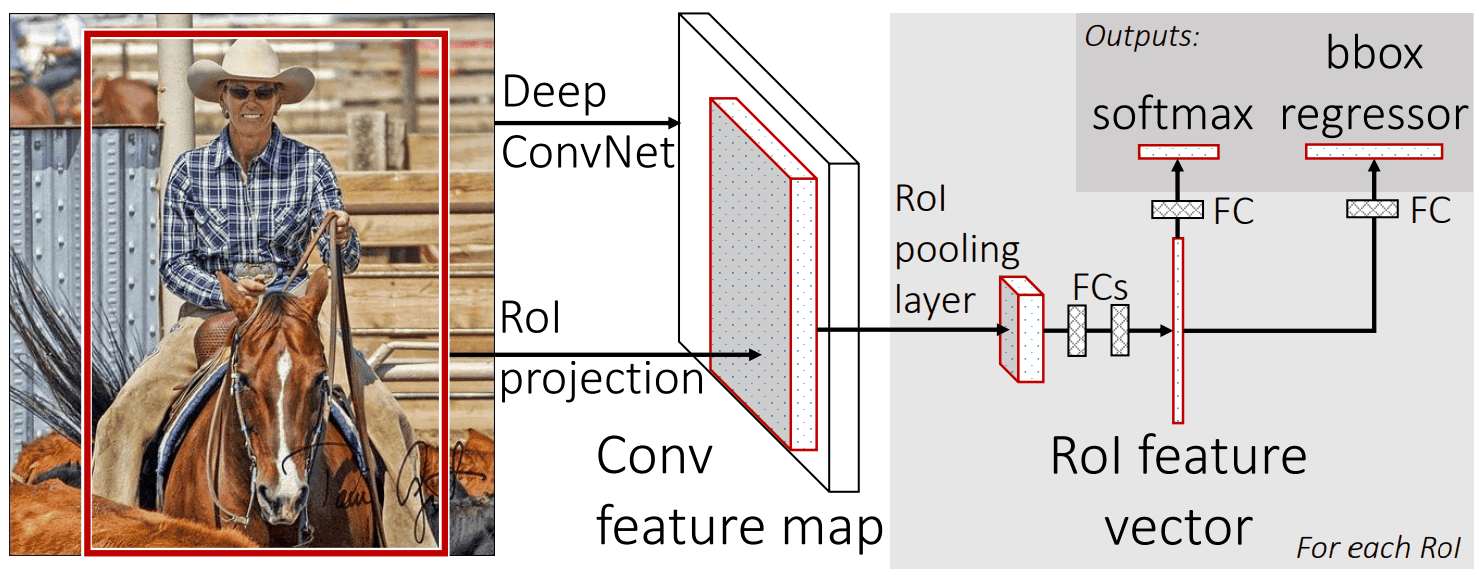## 1. Introduction

In this tutorial, we’ll talk about two computer vision algorithms mainly used for object detection and some of their techniques and applications. Mainly, we’ll walk through the different approaches between R-CNN and Fast R-CNN architecture, and we’ll focus on the ROI pooling layers of Fast R-CNN. Both R-CNN and Fast R-CNN focus on creating bounding boxes in order to recognize and classify the different objects of an image.

## 2. R-CNN Architecture

The architecture of R-CNN looks as follows:The R-CNN neural network was first introduced by Ross Girshick in 2014. As we can see, the authors presented a model that consists of three main tasks. The extraction of region proposals, the computation of the CNN features, and finally the region classification. The algorithm takes an input image and generates a fixed number of approximately 2000 independent region proposals of a fixedpixel size, by using the method of selective search. Then, the network extracts the feature for each generated region by using the deep convolutional neural network that is used to classify images, proposed by Alex Krizhevsky in 2012. The decision on whether the proposed region contains an object or not is made in the last stage by using linear SVMs.

### 2.1. Limitations of R-CNN

Even though R-CNN is a scalable detection algorithm that can achieve a certain precision, there are some disadvantages in its usage. First of all, the number of the region proposals of each image is fixed and can’t be increased. Moreover, the classification of the 2000 regions of each image leads to time-consuming training that cannot be used for real-time applications and tasks. Also, as we mentioned before the algorithm uses selective search in order to produce the 2000 proposed regions. Therefore a poor selection of regions affects the accuracy of the network.

The problems that arose in the R-CNN led to research on its improved version, the Fast R-CNN.

## 3. Fast R-CNN Architecture

The architecture of Fast R-CNN looks as follows:The Fast R-CNN neural network was also introduced by Ross Girshick in 2015. The authors presented an improved model that was able to overcome the limitations of R-CNN and was able to achieve higher accuracy and speed.

As we can see, in this case, a deep fully convolutional network takes as input the entire image and its RoI (Region of Interest) projections, which set the object proposals regions. At first, the network deals with the entire image and generates its feature extraction, a convolutional feature map. This convolutional feature map is responsible for the identification of the proposed regions. Afterward, each RoI is pooled, by the RoI pooling layer and then mapped to a sequence of fully connected layers (FCs). Then, every feature vector, produced by the FCs, is driven into two output layers in order to decide the class of the proposed region. The outputs of the network per RoI are the softmax probabilities and the regression offsets for the bounding box for each class.

The Fast R-CNN algorithm outperforms R-CNN  because the feature extraction takes place once per image, in order for the RoI projections to be generated, instead of performing a convolution forward pass for each object proposal per image, in the case of R-CNN.

## 4. RoI Pooling Layers

First of all, in the Fast R-CNN architecture a Fully Connected Layer, with a fixed size follows the RoI pooling layer. Therefore, because the RoI windows are of different sizes, a pooling technique needs to be applied to them.

The RoI pooling layer, a Spatial pyramid Pooling (SPP) technique is the main idea behind Fast R-CNN and the reason that it outperforms R-CNN in accuracy and speed respectively. SPP is a pooling layer method that aggregates information between a convolutional and a fully connected layer and cuts out the fixed-size limitations of the network. Therefore, the disadvantage of the fixed image size needs is overpassed.

In general, an RoI is a rectangular window and is defined byandvalues that correspond to each window’s top-left corner, height, and width respectively. RoI pooling layers divide arectangular window intoset of sub-windows, and afterward perform max-pooling in each sub-window. The RoI pooling layer performs a max pooling operation in any proposed RoI of an image individually.

Note that the authors of Fast R-CNN propose the value of 7 for H and W.

## 5. Applications

Object detection algorithms can be applied in a wide variety of applications. Both R-CNN and Fast R-CNN algorithms are suitable for creating bounding boxes, counting different items of an image, and separating, and detecting objects within an image which can be really helpful in our lives.

They can be used in a wide variety of real-world applications, like autonomous driving, in order to recognize cars, pedestrians traffic signs, and more, like healthcare for tumor detection and also for recognizing objects in agricultural environments.

## 6. Conclusion

In this article, we walked through R-CNN and Fast R-CNN, object detection algorithms. In particular, we discussed in detail their model architectures and their different approach. We mainly focused on RoI pooling layers and their impact on the speed and accuracy of Fast R-CNN. We also mentioned the applications that these models can be used for in real-life problems.